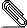[HACKERS] Removing useless DISTINCT clauses

```In  we made a change to process the GROUP BY clause to remove any
group by items that are functionally dependent on some other GROUP BY
items.```
```
This really just checks if a table's PK columns are entirely present
in the GROUP BY clause and removes anything else belonging to that
table.

All this seems to work well, but I totally failed to consider that the
exact same thing applies to DISTINCT too.

Over in , Rui Liu mentions that the planner could do a better job
for his case.

Using Rui Liu's example:

CREATE TABLE test_tbl ( k INT PRIMARY KEY, col text);
INSERT into test_tbl select generate_series(1,10000000), 'test';

Master:

postgres=# explain analyze verbose select distinct col, k from
test_tbl order by k limit 1000;
QUERY PLAN
-------------------------------------------------------------------------------------------------------------------------------------------------
Limit  (cost=1658556.19..1658563.69 rows=1000 width=9) (actual
time=8934.962..8935.495 rows=1000 loops=1)
Output: col, k
->  Unique  (cost=1658556.19..1733557.50 rows=10000175 width=9)
(actual time=8934.961..8935.460 rows=1000 loops=1)
Output: col, k
->  Sort  (cost=1658556.19..1683556.63 rows=10000175 width=9)
(actual time=8934.959..8935.149 rows=1000 loops=1)
Output: col, k
Sort Key: test_tbl.k, test_tbl.col
Sort Method: external merge  Disk: 215128kB
->  Seq Scan on public.test_tbl  (cost=0.00..154056.75
rows=10000175 width=9) (actual time=0.062..1901.728 rows=10000000
loops=1)
Output: col, k
Planning time: 0.092 ms
Execution time: 8958.687 ms
(12 rows)

Patched:

postgres=# explain analyze verbose select distinct col, k from
test_tbl order by k limit 1000;

QUERY PLAN
----------------------------------------------------------------------------------------------------------------------------------------------------------
Limit  (cost=0.44..34.31 rows=1000 width=9) (actual time=0.030..0.895
rows=1000 loops=1)
Output: col, k
->  Unique  (cost=0.44..338745.50 rows=10000175 width=9) (actual
time=0.029..0.814 rows=1000 loops=1)
Output: col, k
->  Index Scan using test_tbl_pkey on public.test_tbl
(cost=0.44..313745.06 rows=10000175 width=9) (actual time=0.026..0.452
rows=1000 loops=1)
Output: col, k
Planning time: 0.152 ms
Execution time: 0.985 ms
(8 rows)

A patch to implement this is attached.

I'll add it to the Jan commitfest. (I don't expect anyone to look at
this before then).


https://git.postgresql.org/gitweb/?p=postgresql.git;a=commit;h=d4c3a156cb46dcd1f9f97a8011bd94c544079bb5


https://www.postgresql.org/message-id/flat/CAKJS1f9q0j3BgMUsDbtf9%3DecfVLnqvkYB44MXj0gpVuamcN8Xw%40mail.gmail.com#CAKJS1f9q0j3BgMUsDbtf9=ecfvlnqvkyb44mxj0gpvuamcn...@mail.gmail.com

--remove_useless_distinct_clauses.patch
```--